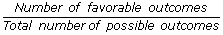## Definition Of Probability

Probability is a numerical measure of the likelihood of occurrence of an event. The value of probability lies between 0 and 1.

If all outcomes of an experiment are equally likely, then the probability is given by, Probability of an event =### Example of Probability

The probability to pick a blue marble from a basket containing 10 blue marbles is 1.
Suppose you toss a fair coin. Then the probability of tossing a head or tail is 1/2

A. 1/3
B.1/2
C.1/6
D.1/5